# Wyatt Research Group Movies

The focus of our research has been energy flow in molecules. All of the animations here represent trajectories that were calculated at the state of the art in computational chemistry. Most of the animations were created to aid the research group in visualizing the motion represented by the calculations. Unless otherwise noted, all of the computations were performed by Todd J. Minehardt, Robert E. Wyatt, and J. David Adcock using lonestar, a Cray T3E Massively Parallel Computer system. All of these animations were produced by J. David Adcock. The graphics were rendered using the POV Ray Version 3.0.1 program.

### Quasi-Classical Dynamics of the Benzene Molecule

The first set of animations were made to illustrate the vibrational dynamics of the benzene molecule, C6H6. All of the animations of these studies are based on computations using the most extensive ab initioforce field available for a polyatomic molecule, published by Maslen, Handy, Amos, and Jayatilaka1 in 1992. The original force field contains terms through the fourth-order, and these terms have been supplemented by fifth- and sixth-order terms by Wyatt and Iung2.

In each of the studies, we investigate the quasi-classical dynamics of benzene by computing many possible trajectories of the molecule under varying initial conditions and with various constraints. Each animation represents one possible trajectory of the molecule. Several different types of animations are presented.

In the animation at top right, the trajectory of the molecule is constrained to a plane (i.e., 21-mode benzene3). In the other two animations, out-of-plane motion is allowed (i.e., 30-mode benzene4,5). The total duration of each of the full simulations is 2.4 picoseconds, though the short example animations below represent only small fractions of this interval. In these animations, the small blue spheres represent the hydrogen atoms, and the red spheres represent the carbon atoms.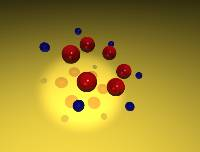A Carbon's Eye View
of Molecular Dynamics
in Benzene

QuickTime
2.2 Mb.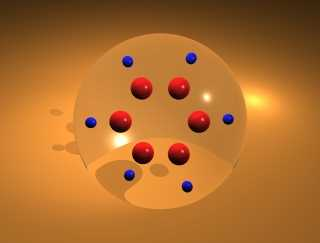Molecular Dynamics
of Benzene, 30 mode
First overtone

QuickTime
5.7 Mb.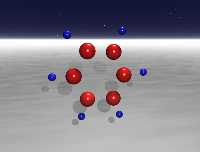Molecular Dynamics
of Benzene, 30 mode
Second overtone

QuickTime
3.2 Mb.

### The C-H Chromophore in C6H

This set of animations were made to study the (quasi-classical) dynamics of the C-H Chromophore in C6H. This study6 is a (15-mode) subset of the full benzene problem and was done to determine the scalability of certain elements of the calculations of the full benzene problem. In these animations, only the C-H chromophore is shown, and the yellow sphere represents the hydrogen atom, while the blue sphere represents the carbon atom. In order to emphasize the motion of the hydrogen atom, the yellow sphere is plotted at the successive locations of the hydrogen atom in the model, and the preceeding locations of the atom are shown by an orange track.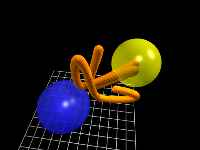C-H chromophore
motion in C6H

QuickTime
6.0 Mb.

### Quantum Dynamics of (30-mode) Benzene

This set of animations depicts a study of the quantum dynamics of the benzene7 molecule. Since this is a quantum study, the Cartesian positions of the atoms in the molecule are not readily available. Instead, we illustrate the energy levels of the six classes of local mode vibration:
• C-H Stretch
• C-C Stretch
• In-plane C-H Wag
• C-C-C Bend
• Out-of-plane C-H Bend
• C-C-C-C Ring Torsion
during the first 100 femtoseconds after excitation.
•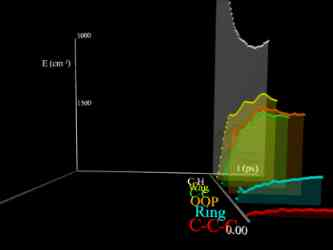Quantum Dynamics
of 30-mode Benzene

QuickTime
4.6 Mb.

### Quantum Trajectory Method

The next class of animation depicts a sample application of the Quantum Trajectory Method (QTM). This study follows the progress of a reaction of the form
A + BC -> AB + C
in the reaction coordinate.

QTM treats regions of probability density as fluid "particles" and applies the principles of fluid dynamics to determine the motion of these "particles".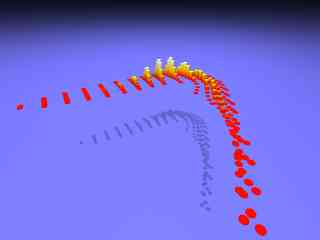Quantum Trajectory
Method

QuickTime
3.0 Mb.

### Quasiclassical Trajectory (QCT) Calculations for O + HCl -> OH + Cl

The next animation depicts the chemical reaction

HCl + O -> Cl + HO

as computed by Quasi-Classical Trajectory (QCT) calculations. The computations were done by Bala Ramachandran8 at Louisiana Tech University.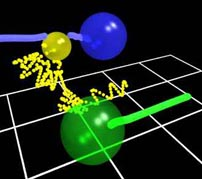Quasiclassical
Trajectory (QCT)
calculation for
O + HCl -> OH + Cl

QuickTime
6.0 Mb.

References
1. P.E. Maslen, N.C. Handy, R.D. Amos, and D. Jayatilaka, J. Chem. Phys. 97, 4233 (1992).
2. R.E. Wyatt and C. Iung, J. Chem. Phys. 98, 6758 (1993).
3. T.J.Minehardt and R.E. Wyatt, J. Chem. Phys. 109, 8330 (1998).
4. T.J.Minehardt and R.E. Wyatt, Chem. Phys. Lett. 295, 373 (1998).
5. T.J.Minehardt, J.D. Adcock, and R.E. Wyatt, Chem. Phys. Lett. 303, 537 (1999).
6. T.J. Minehardt, J.D. Adcock, and R.E. Wyatt, Chem. Phys. Lett. 303, 347 (1999).
7. T.J.Minehardt and R.E. Wyatt, J. Chem. Phys. 110, 3326 (1999).
8. B. Ramachandran, E.A. Schrader III, J. Senekowitsch, and R.E. Wyatt, J. Chem. Phys. 111, 3862 (1999).